### Home > CALC > Chapter 4 > Lesson 4.1.3 > Problem4-33

4-33.
1.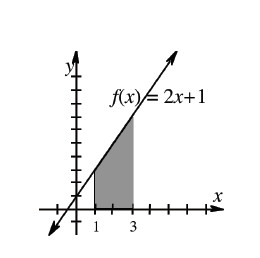Given the graph to the right of f(x) = 2x + 1, find: Homework Help ✎

1.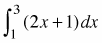2.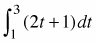3. What is the difference between the expressions in parts (a) and (b)?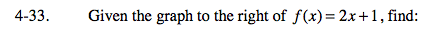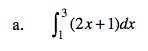It's a trapezoid!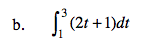You could sketch another graph where the horizontal axis is called t. Or you could let x = t and use the graph above.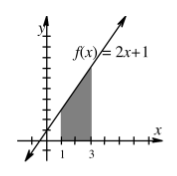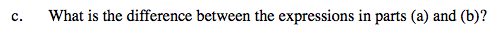None. The finite areas are the same.